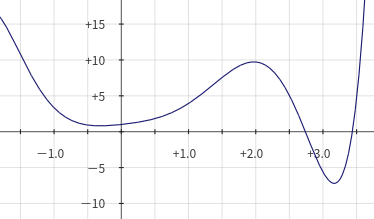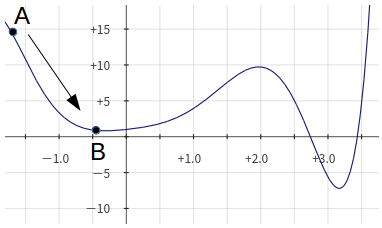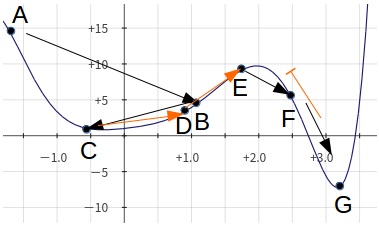# 算法简述## 实现过程

1. $T$——温度
2. $\Delta T$——温度变化率，每次温度等于上一次温度乘上$\Delta T$，实际应用时一般取$0.95-0.99$，模拟徐徐降温

1. $x$——当前选择的解
2. $\Delta x$——解变动值
3. $x_1$——当前的目标解，等于$x+\Delta x$
4. $\Delta f$——当前解的函数值与目标解函数值之差，等于$f(x)-f(x_1)$## 关于参数

update:考试的时候写提答，总结了一种方法：观察法

# 更新中！

posted @ 2018-04-19 16:36  Flash_Hu  阅读(34824)  评论(20编辑  收藏